Chapter 6 Functions Topics Basics Simplest functions Functions

• Slides: 51Chapter 6 Functions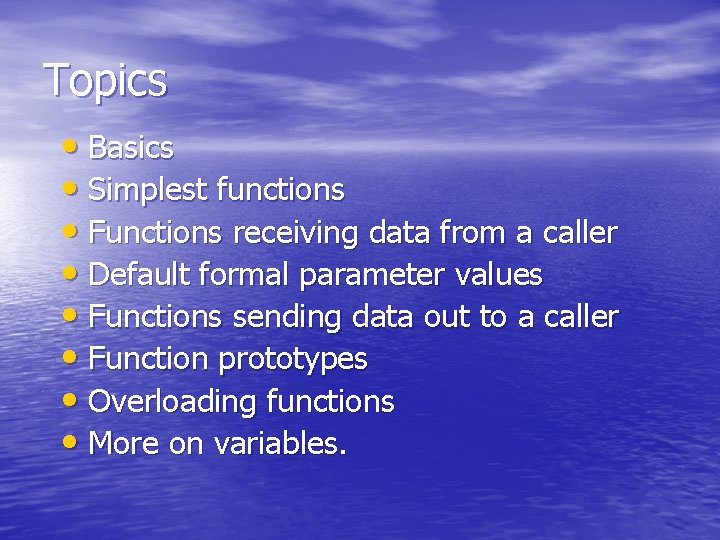Topics • Basics • Simplest functions • Functions receiving data from a caller • Default formal parameter values • Functions sending data out to a caller • Function prototypes • Overloading functions • More on variables.Modular Programming • Modular programming: breaking a program up into smaller, manageable functions or modules • Function: a collection of statements to perform a task • Motivation for modular programming: – Improves maintainability of programs – Simplifies the process of writing programs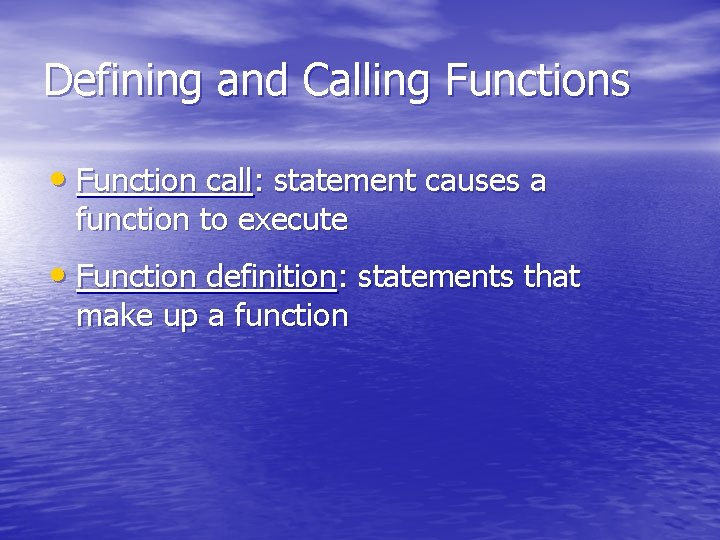Defining and Calling Functions • Function call: statement causes a function to execute • Function definition: statements that make up a function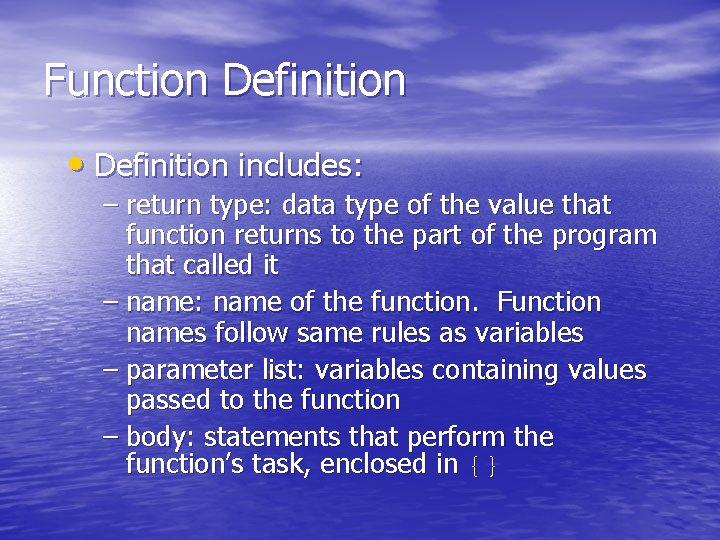Function Definition • Definition includes: – return type: data type of the value that function returns to the part of the program that called it – name: name of the function. Function names follow same rules as variables – parameter list: variables containing values passed to the function – body: statements that perform the function’s task, enclosed in {}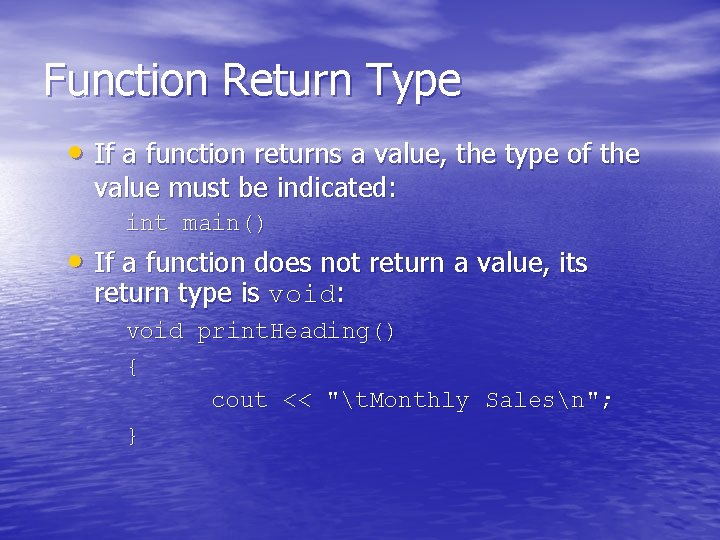Function Return Type • If a function returns a value, the type of the value must be indicated: int main() • If a function does not return a value, its return type is void: void print. Heading() { cout << "t. Monthly Salesn"; }Calling a Function • To call a function, use the function name followed by () and ; print. Heading(); • When called, program executes the body of the called function • After the function terminates, execution resumes in the calling function at point of call.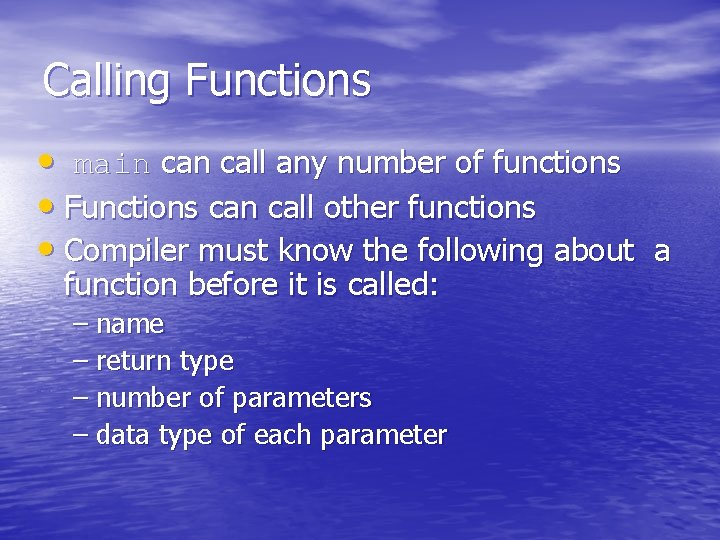Calling Functions • main call any number of functions • Functions can call other functions • Compiler must know the following about a function before it is called: – name – return type – number of parameters – data type of each parameter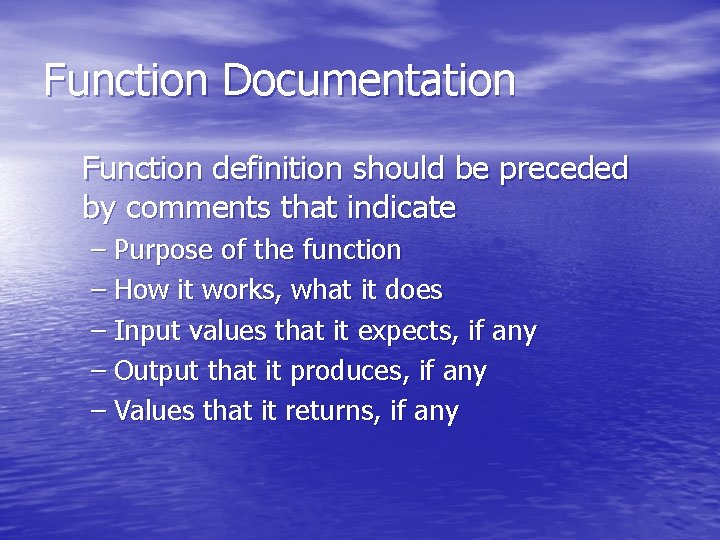Function Documentation Function definition should be preceded by comments that indicate – Purpose of the function – How it works, what it does – Input values that it expects, if any – Output that it produces, if any – Values that it returns, if any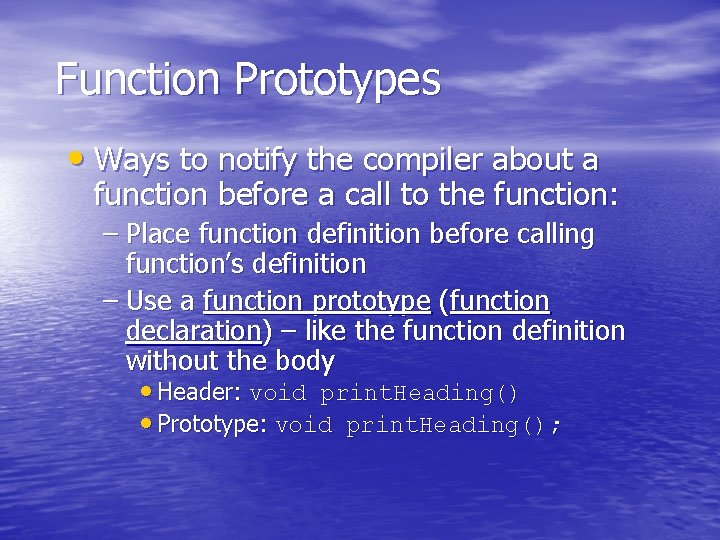Function Prototypes • Ways to notify the compiler about a function before a call to the function: – Place function definition before calling function’s definition – Use a function prototype (function declaration) – like the function definition without the body • Header: void print. Heading() • Prototype: void print. Heading();Prototype Notes • Place prototypes near top of program • Program must include either prototype or full function definition before any call to the function – compiler error otherwise • When using prototypes, can place function definitions in any order in source fileExample #include <iostream> using namespace std; //function declarations void first(); void second(); int main() { } cout << “I am starting in function” <<“main. n”; first(); second(); cout << “back in function main again n”; return 0; //Definition of function first //this function displays a message void first() { cout << “I am now inside the” << “function first n”; } //Definition of function second //this function displays a message void second() { cout << “I am now inside the” << “function second n”; }Sending Data into a Function • Can pass values into a function at time of call: c = sqrt(a*a + b*b); • Values passed to function are arguments • Variables in function that hold values passed as arguments are parametersOther Parameter Terminology • A parameter can also be called a formal parameter or a formal argument • An argument can also be called an actual parameter or an actual argument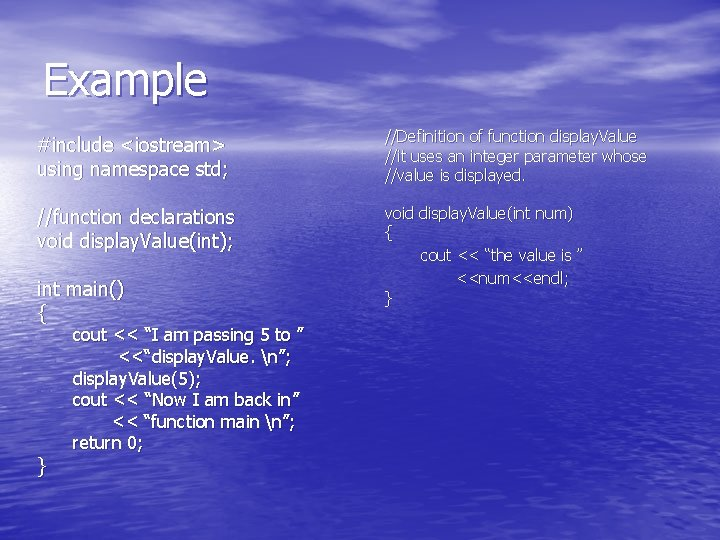Example #include <iostream> using namespace std; //Definition of function display. Value //it uses an integer parameter whose //value is displayed. //function declarations void display. Value(int); void display. Value(int num) { cout << “the value is ” <<num<<endl; } int main() { } cout << “I am passing 5 to ” <<“display. Value. n”; display. Value(5); cout << “Now I am back in” << “function main n”; return 0;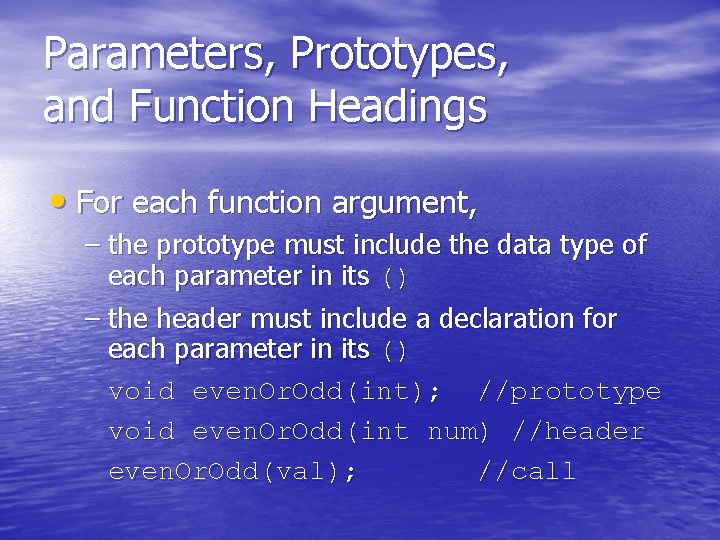Parameters, Prototypes, and Function Headings • For each function argument, – the prototype must include the data type of each parameter in its () – the header must include a declaration for each parameter in its () void even. Or. Odd(int); //prototype void even. Or. Odd(int num) //header even. Or. Odd(val); //call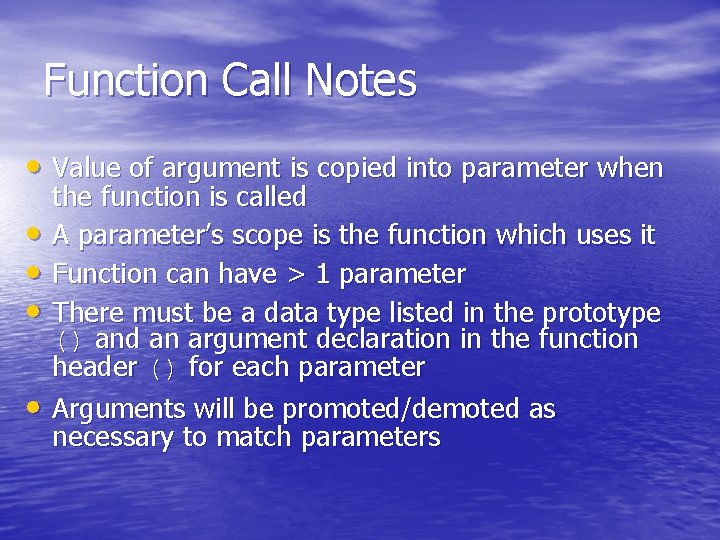Function Call Notes • Value of argument is copied into parameter when • • the function is called A parameter’s scope is the function which uses it Function can have > 1 parameter There must be a data type listed in the prototype () and an argument declaration in the function header () for each parameter Arguments will be promoted/demoted as necessary to match parameters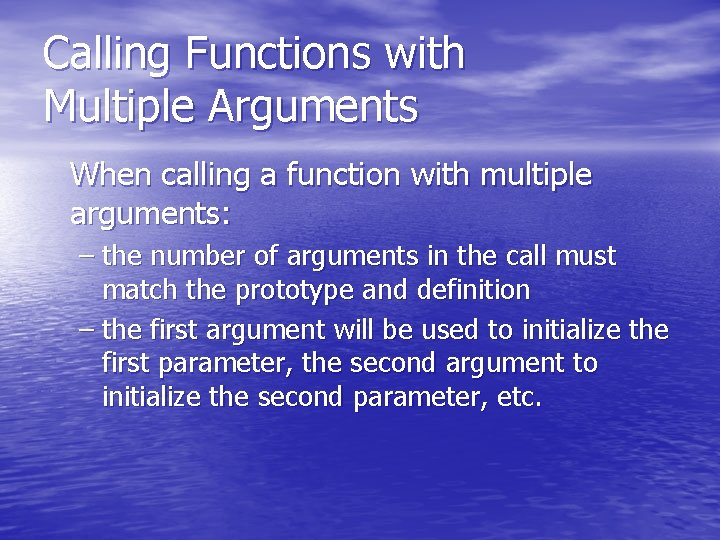Calling Functions with Multiple Arguments When calling a function with multiple arguments: – the number of arguments in the call must match the prototype and definition – the first argument will be used to initialize the first parameter, the second argument to initialize the second parameter, etc.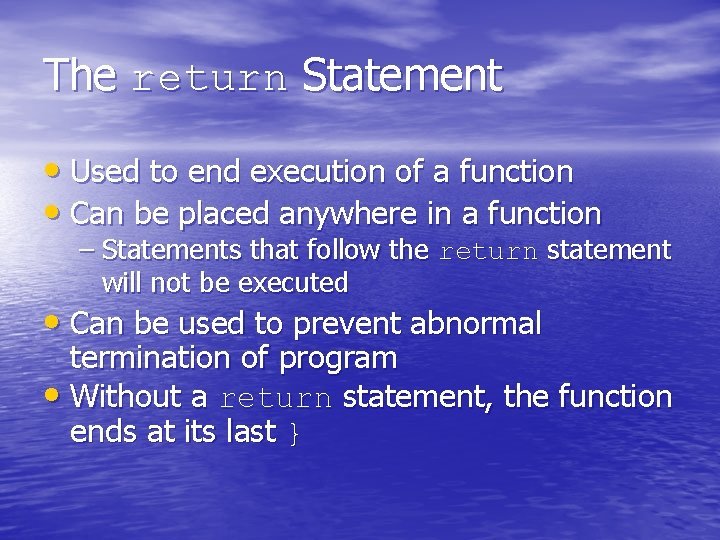The return Statement • Used to end execution of a function • Can be placed anywhere in a function – Statements that follow the return statement will not be executed • Can be used to prevent abnormal termination of program • Without a return statement, the function ends at its last }Example #include <iostream> using namespace std; //function declarations void divide(double, double); int main() { double num 1, num 2; } cout << “Enter two numbers and I will divide ” << “the first number by the second n”; cin >> num 1 >> num 2; divide ( num 1, num 2); return 0; //Definition of function divide //uses two parameters: arg 1 and arg 2. the // function divides arg 1 by arg 2 and shows // the results. If arg 2 is zero, the function //returns void divide (double arg 1, double arg 2) { If (arg 2 == 0) { cout << “Sorry, I cannot divide by” << “zero n”; return; } cout<< “the result is ” << (arg 1/arg 2) <<endl; }Returning a Value From a Function • return statement can be used to return a value from function to the point of call • Prototype and definition must indicate data type of return value (not void) • Calling function should use return value: – assign it to a variable – send it to cout – use it in an expression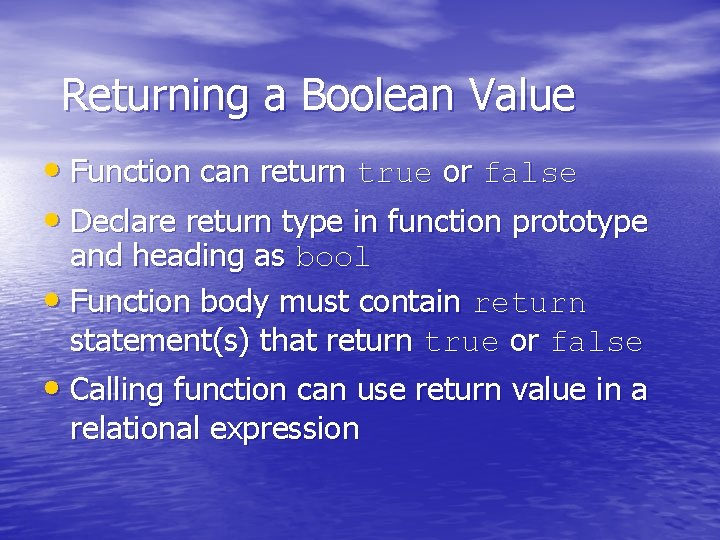Returning a Boolean Value • Function can return true or false • Declare return type in function prototype and heading as bool • Function body must contain return statement(s) that return true or false • Calling function can use return value in a relational expression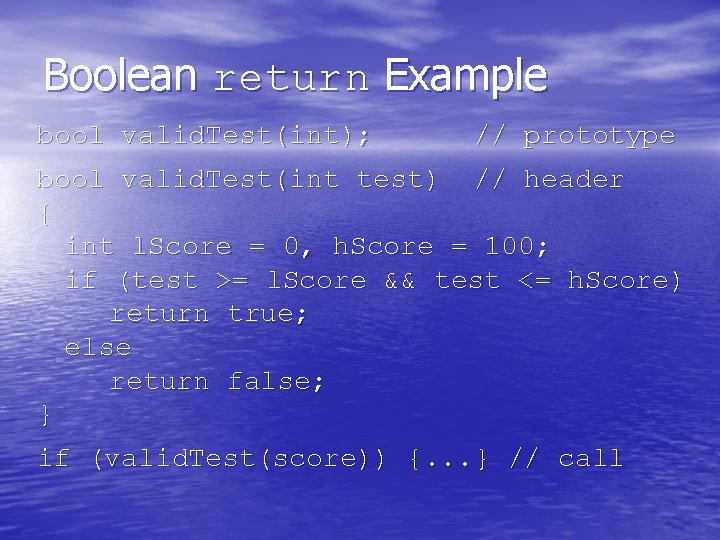Boolean return Example bool valid. Test(int); // prototype bool valid. Test(int test) // header { int l. Score = 0, h. Score = 100; if (test >= l. Score && test <= h. Score) return true; else return false; } if (valid. Test(score)) {. . . } // callPassing Data by Value • Pass by value: when an argument is passed to a function, its value is copied into the parameter. • Changes to the parameter in the function do not affect the value of the argumentPassing Information to Parameters by Value • Example: int val=5; even. Or. Odd(val); val 5 argument in calling function num 5 parameter in even. Or. Odd function • even. Or. Odd can change variable num, but it will have no effect on variable valExample #include <iostream> using namespace std; //function declarations void change. Them(int, double); int main() { int whole=12; double real=3. 5; } cout << “the value of whole is ” << whole <<“n”; cout << “the value of real is ” << real <<“n”; change. Them ( whole, real); cout << “Now, the value of whole is ” << whole <<“n”; cout << “the value of real is ” << real <<“n”; return 0; //Definition of function change. Them //it uses I, an int parameter, and f, a // double, the values of i and f are // changed and displayed. void change. Them(int i, double f) { i=100; f=24. 5; cout << “value of i is changed to” << i <<“n”; cout << “value of f is changed to” << f <<“n”; }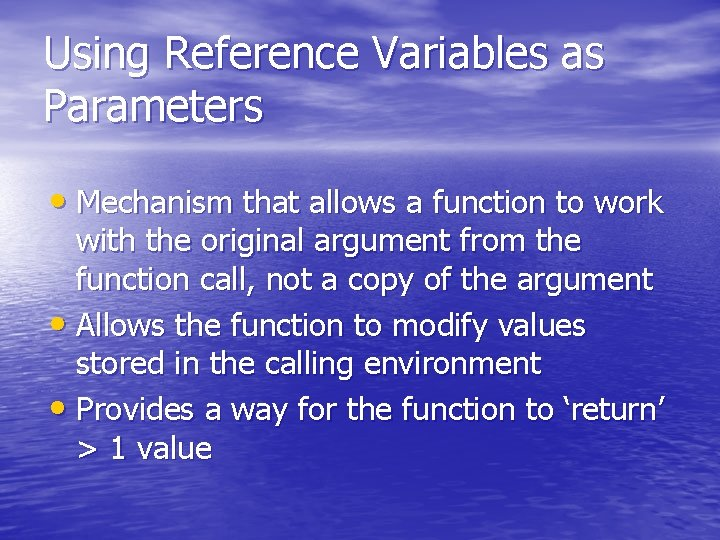Using Reference Variables as Parameters • Mechanism that allows a function to work with the original argument from the function call, not a copy of the argument • Allows the function to modify values stored in the calling environment • Provides a way for the function to ‘return’ > 1 value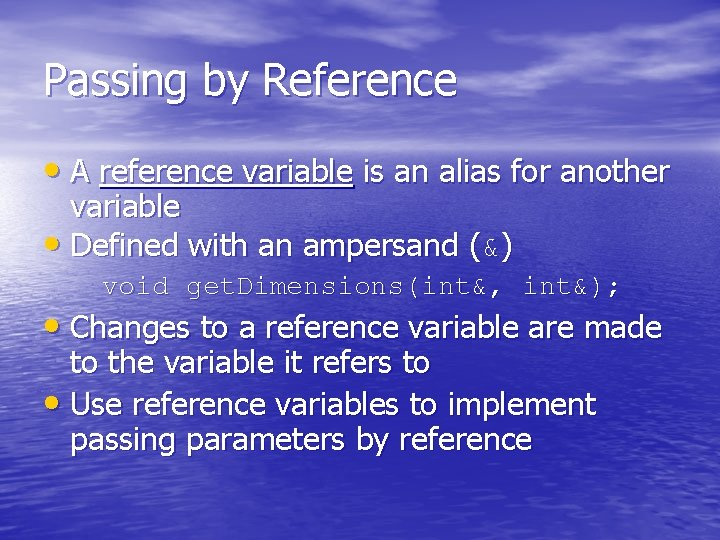Passing by Reference • A reference variable is an alias for another variable • Defined with an ampersand (&) void get. Dimensions(int&, int&); • Changes to a reference variable are made to the variable it refers to • Use reference variables to implement passing parameters by reference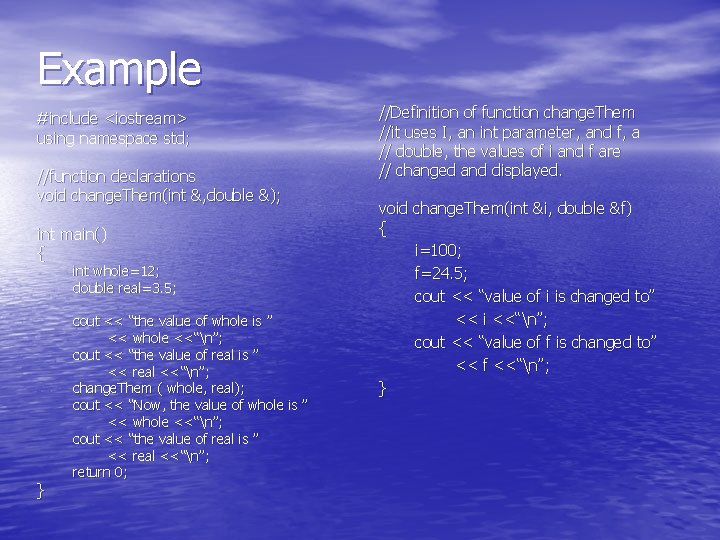Example #include <iostream> using namespace std; //function declarations void change. Them(int &, double &); int main() { int whole=12; double real=3. 5; } cout << “the value of whole is ” << whole <<“n”; cout << “the value of real is ” << real <<“n”; change. Them ( whole, real); cout << “Now, the value of whole is ” << whole <<“n”; cout << “the value of real is ” << real <<“n”; return 0; //Definition of function change. Them //it uses I, an int parameter, and f, a // double, the values of i and f are // changed and displayed. void change. Them(int &i, double &f) { i=100; f=24. 5; cout << “value of i is changed to” << i <<“n”; cout << “value of f is changed to” << f <<“n”; }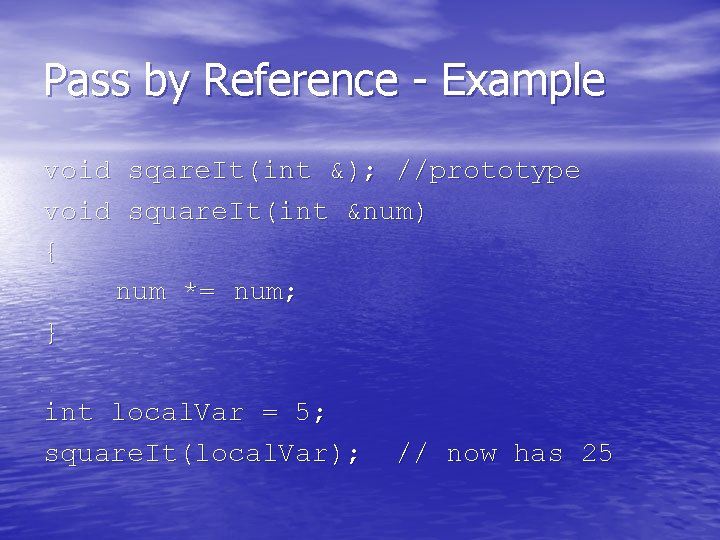Pass by Reference - Example void sqare. It(int &); //prototype void square. It(int &num) { num *= num; } int local. Var = 5; square. It(local. Var); // now has 25Reference Variable Notes • Each reference parameter must contain & • Space between type and & is unimportant • Must use & in both prototype and header • Argument passed to reference parameter must • be a variable – cannot be an expression or constant Use when appropriate – don’t use when argument should not be changed by function, or if function needs to return only 1 valueUsing Functions in Menu-Driven Programs • Functions can be used – to implement user choices from menu – to implement general-purpose tasks: • Higher-level functions can call general-purpose functions, minimizing the total number of functions and speeding program development time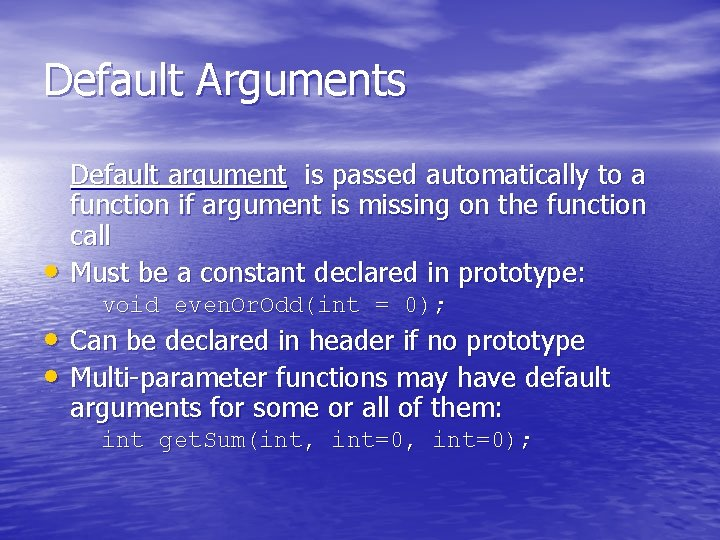Default Arguments • Default argument is passed automatically to a function if argument is missing on the function call Must be a constant declared in prototype: void even. Or. Odd(int = 0); • Can be declared in header if no prototype • Multi-parameter functions may have default arguments for some or all of them: int get. Sum(int, int=0);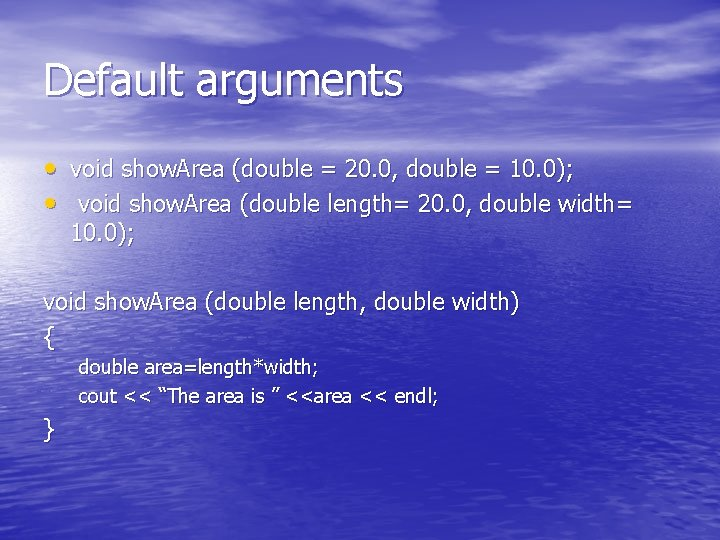Default arguments • void show. Area (double = 20. 0, double = 10. 0); • void show. Area (double length= 20. 0, double width= 10. 0); void show. Area (double length, double width) { double area=length*width; cout << “The area is ” <<area << endl; }Default arguments void show. Area (double length = 20. 0, double width = 10. 0) { double area=length*width; cout << “The area is ” <<area << endl; }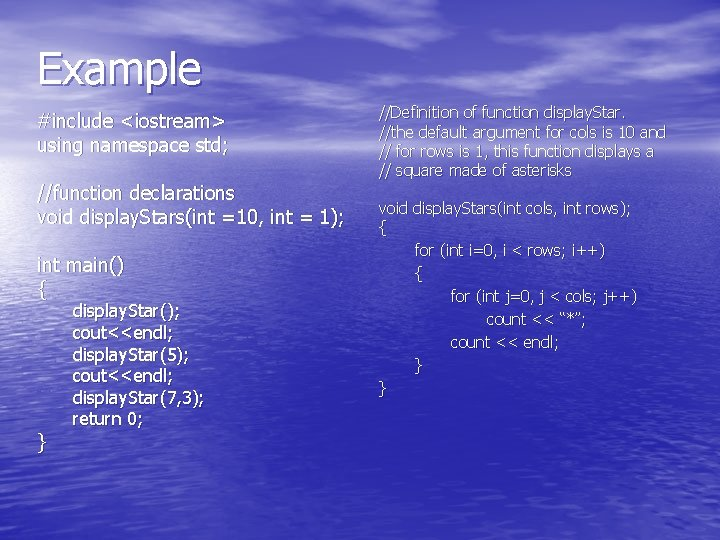Example #include <iostream> using namespace std; //function declarations void display. Stars(int =10, int = 1); int main() { } display. Star(); cout<<endl; display. Star(5); cout<<endl; display. Star(7, 3); return 0; //Definition of function display. Star. //the default argument for cols is 10 and // for rows is 1, this function displays a // square made of asterisks void display. Stars(int cols, int rows); { for (int i=0, i < rows; i++) { for (int j=0, j < cols; j++) count << “*”; count << endl; } }Default arguments • If not all parameters to a function have default values, the defaultless ones are declared first in the parameter list: int get. Sum(int, int=0); int get. Sum(int num 1, int num 2, int num 3) { int sum; sum = num 1+num 2+num 3; cout << “the sum of three number is ”<<sum<<endl; return sum; } int get. Sum(int, int=0, int); // int get. Sum(int=0, int); //Local variable vs. Global Varialbe • The general structure of a program: . . . Type 1 function 1(…) {…}. . . Typen functionn (…) {…} int main () {…}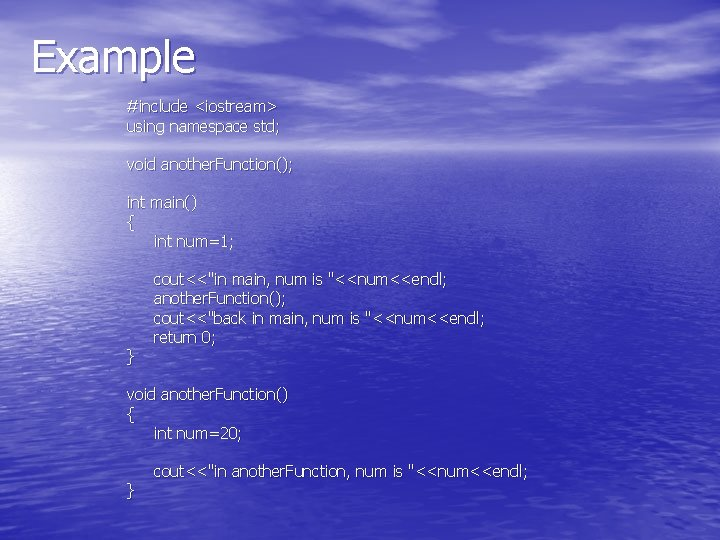Example #include <iostream> using namespace std; void another. Function(); int main() { int num=1; } cout<<"in main, num is "<<num<<endl; another. Function(); cout<<"back in main, num is "<<num<<endl; return 0; void another. Function() { int num=20; } cout<<"in another. Function, num is "<<num<<endl;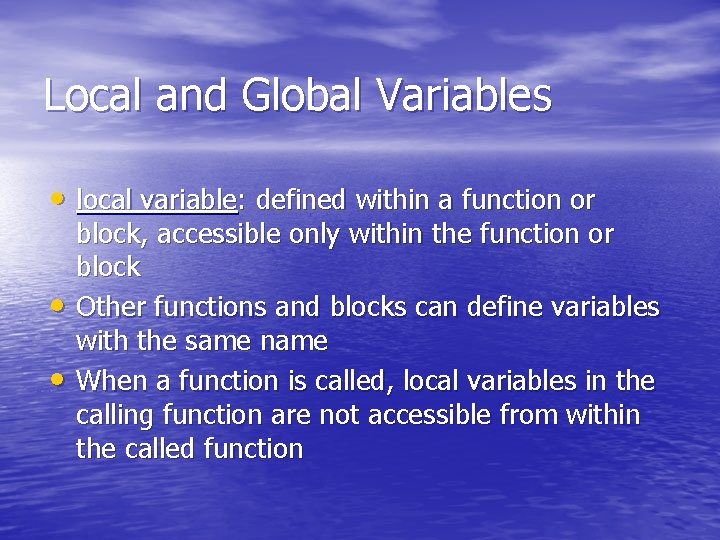Local and Global Variables • local variable: defined within a function or • • block, accessible only within the function or block Other functions and blocks can define variables with the same name When a function is called, local variables in the calling function are not accessible from within the called function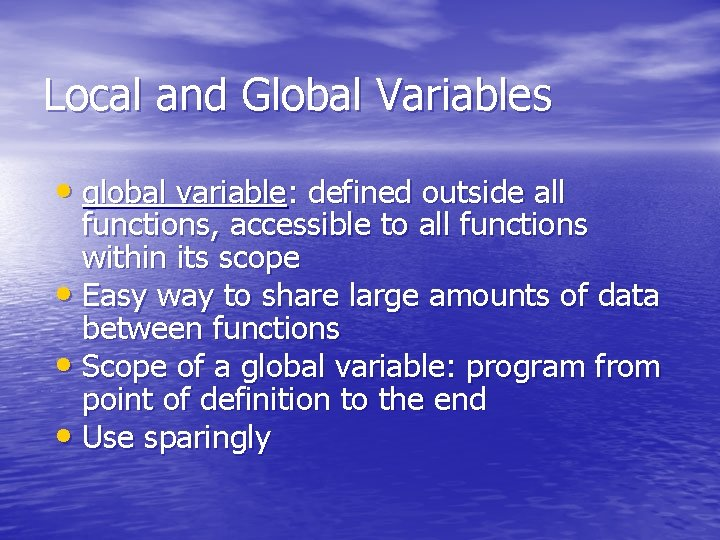Local and Global Variables • global variable: defined outside all functions, accessible to all functions within its scope • Easy way to share large amounts of data between functions • Scope of a global variable: program from point of definition to the end • Use sparinglyExample #include <iostream> using namespace std; void another. Function(); int num=1; int main() { cout<<"in main, num is "<<num<<endl; another. Function(); num=70; cout<<"back in main, num is "<<num<<endl; return 0; } void another. Function() { cout<<"in another. Function, num is "<<num<<endl; num=20; cout<<"in another. Function, num is "<<num<<endl; }Initializing Local and Global Variables • Local variables must be initialized by programmer • Global variables are initialized to 0 (numeric) or NULL (character) when variable is defined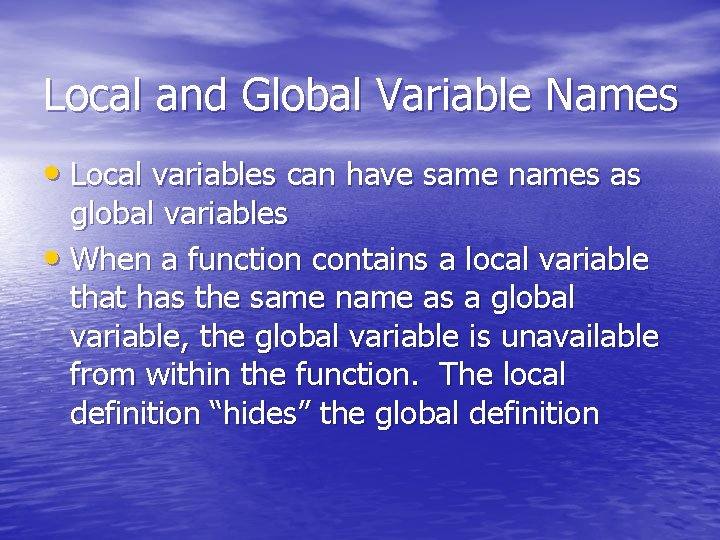Local and Global Variable Names • Local variables can have same names as global variables • When a function contains a local variable that has the same name as a global variable, the global variable is unavailable from within the function. The local definition “hides” the global definition#include <iostream> using namespace std; void springfield(); void Qin. Dao(); int students=20000; int main() { cout<<“there are "<<students<<“ students”<<endl; springfield(); void Qin. Dao(); cout<<"back in main, students "<<students<<endl; return 0; } void springfield() { int students=19000; cout<<"in springfield, "<<students<<endl; } void Qin. Dao() { int students=1000; cout<<"in Qin. Dao, "<<students<<endl; }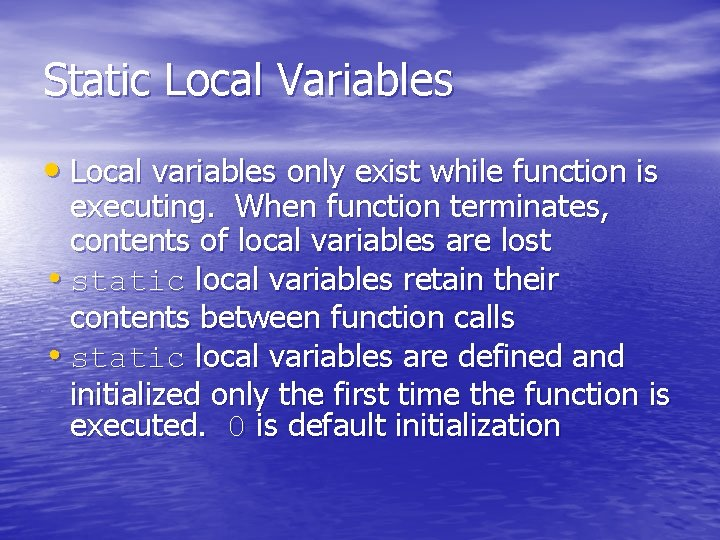Static Local Variables • Local variables only exist while function is executing. When function terminates, contents of local variables are lost • static local variables retain their contents between function calls • static local variables are defined and initialized only the first time the function is executed. 0 is default initializationOverloading Functions • Overloaded functions have the same name • • but different parameter lists Can be used to create functions that perform the same task but take different parameter types or different number of parameters Compiler will determine which version of function to call by argument and parameter lists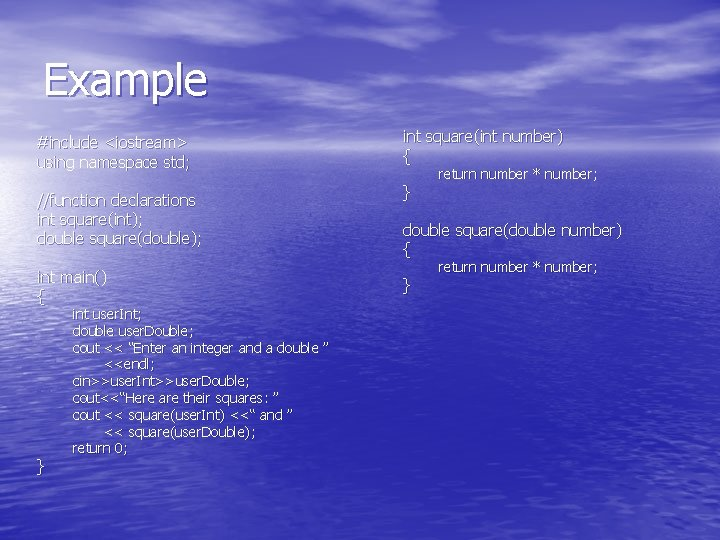Example #include <iostream> using namespace std; //function declarations int square(int); double square(double); int main() { } int user. Int; double user. Double; cout << “Enter an integer and a double ” <<endl; cin>>user. Int>>user. Double; cout<<“Here are their squares: ” cout << square(user. Int) <<“ and ” << square(user. Double); return 0; int square(int number) { } return number * number; double square(double number) { } return number * number;Function Overloading Examples Using these overloaded functions, void get. Dimensions(int); // get. Dimensions(int, double); // get. Dimensions(double, double); // the compiler will use them as follows: int length, width; double base, height; get. Dimensions(length); get. Dimensions(length, width); get. Dimensions(length, height); get. Dimensions(height, base); // // 1 2 3 4The exit() Function • Terminates execution of a program • Can be called from any function • Can pass an int value to operating system to indicate status of program termination • Usually used for abnormal termination of program • Requires cstdlib header file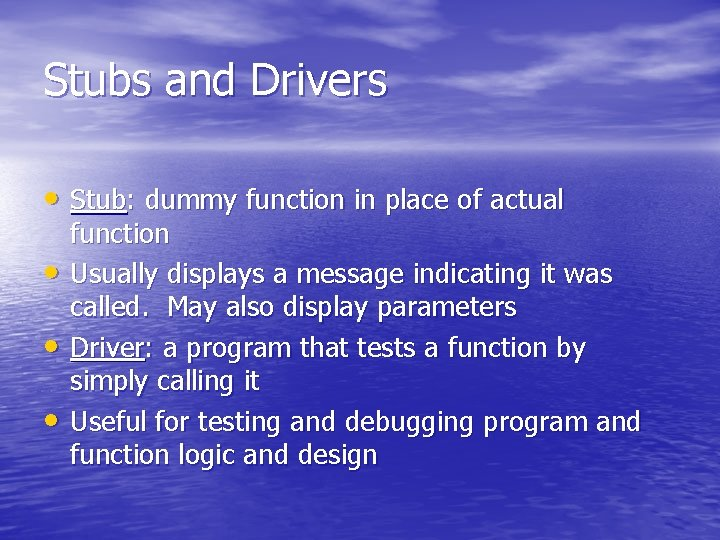Stubs and Drivers • Stub: dummy function in place of actual • • • function Usually displays a message indicating it was called. May also display parameters Driver: a program that tests a function by simply calling it Useful for testing and debugging program and function logic and design